# 局部敏感哈希（LSH）

## 一. 近邻搜索

LSH主要运用到高维海量数据的快速近似查找。近似查找便是比较数据点之间的距离或者是相似度。因此，很明显，LSH是向量空间模型下的东西。一切数据都是以点或者说以向量的形式表现出来的。在细说LSH之前必须先提一下K最近邻查找 (kNN，k-Nearest Neighbor)与c最近邻查找 (cNN，c-Nearest Neighbor )。

kNN问题就不多说了，这个大家应该都清楚，在一个点集中寻找距离目标点最近的K个点。我们主要提一下cNN问题。首先给出最近邻查找（NN，Nearest Neighbor）的定义。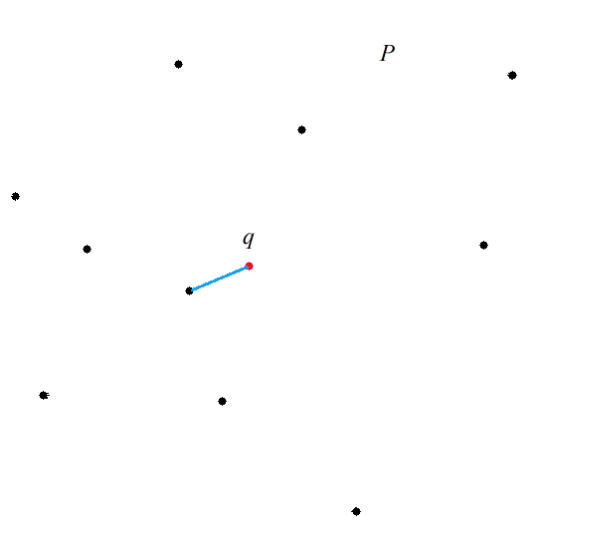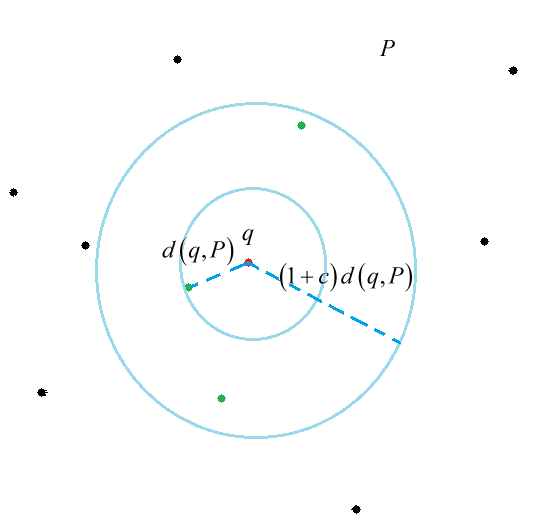cNN不同于kNN，cNN和距离的联系更加紧密。LSH本身设计出来是专门针对解决cNN问题，而不是kNN问题，但是很多时候kNN与cNN有着相似的解集。因此LSH也可以运用在kNN问题上。这些问题若使用一一匹配的暴力搜索方式会消耗大量的时间，即使这个时间复杂度是线性的。

## 二. LSH的定义

LSH不像树形结构的方法可以得到精确的结果，LSH所得到的是一个近似的结果，因为在很多领域中并不需非常高的精确度。即使是近似解，但有时候这个近似程度几乎和精准解一致。

LSH的主要思想是，高维空间的两点若距离很近，那么设计一种哈希函数对这两点进行哈希值计算，使得他们哈希值有很大的概率是一样的。同时若两点之间的距离较远，他们哈希值相同的概率会很小。给出LSH的定义如下：

若  p ∈ B ( q , r 1 )  则  Pr ⁡ H [ h ( q ) = h ( p ) ] ≥ p 1 若  p ∉ B ( q , r 2 )  则  Pr ⁡ H [ h ( q ) = h ( p ) ] ≤ p 2 \begin{array}{l} \text { 若 } p \in B\left(q, r_{1}\right) \text { 则 } \operatorname{Pr}_{H}[h(q)=h(p)] \geq p_{1} \\ \text {若 } p \notin B\left(q, r_{2}\right) \text { 则 } \operatorname{Pr}_{H}[h(q)=h(p)] \leq p_{2} \end{array}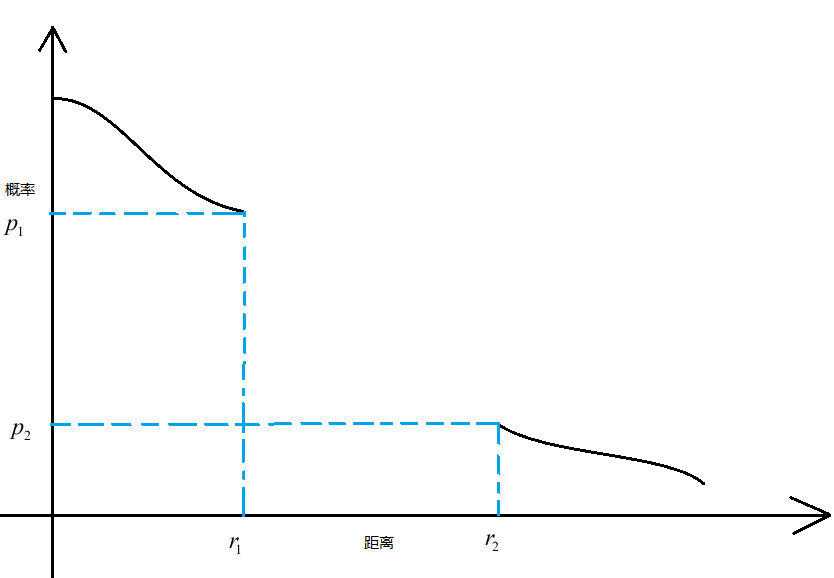## 三. 曼哈顿距离转换成汉明距离

曼哈顿距离又称L1L1范数距离。其具体定义如下：

Manhattan ⁡ ( A , B ) = ∑ i = 1 n ∣ a i − b i ∣ \operatorname{Manhattan}(A, B)=\sum_{i=1}^{n}\left|a_{i}-b_{i}\right|

1.使用L1范数距离进行度量。
2.所有坐标全部被正整数化。
对于第一条他解释说L1范数距离与L2范数距离在进行近似查找时得到的结果非常相似。对于第二条，整数化是为了方便进行01编码。

v ( p ) = Unaryc ⁡ ( x 1 ) Unaryc ⁡ ( x 2 ) … Unaryc ⁡ ( x n ) v(p)=\operatorname{Unaryc}\left(x_{1}\right) \operatorname{Unaryc}\left(x_{2}\right) \ldots \operatorname{Unaryc}\left(x_{n}\right)
Unary_ ( x ) (x) 是一串长度为 C C 二进制的汉明码，其意思是前 位为1 后 位为0。举个例子, C若为5 x 为3，则 Unary 5 ( 3 ) _{5}(3) =11100 。 v ( p ) v(p) 是多个 Unary C ( x ) _{C}(x) 拼接而成。此时可以发现对于两点 p , q p, q 他们之间的曼哈顿距离和通过变换坐标后的汉明距 离是一样的。到此处, 我们可以针对汉明距离来定义一族哈希函数。

##四. 汉明距离下的LSH哈希函数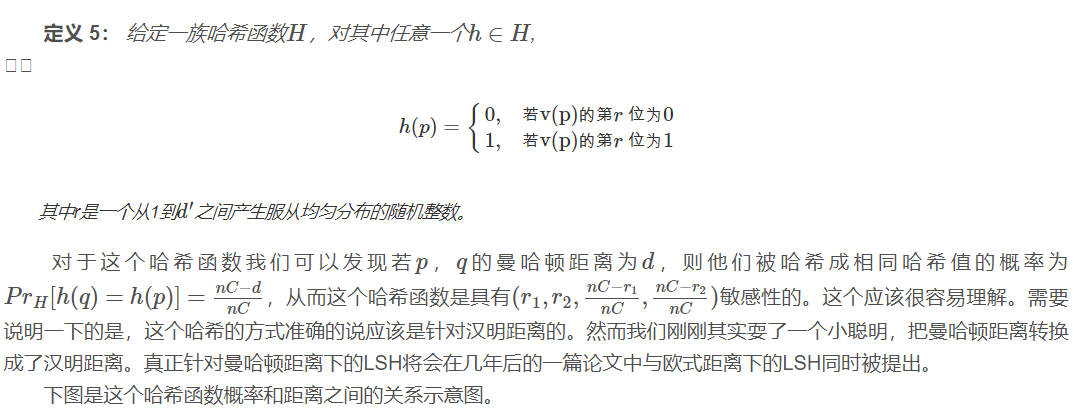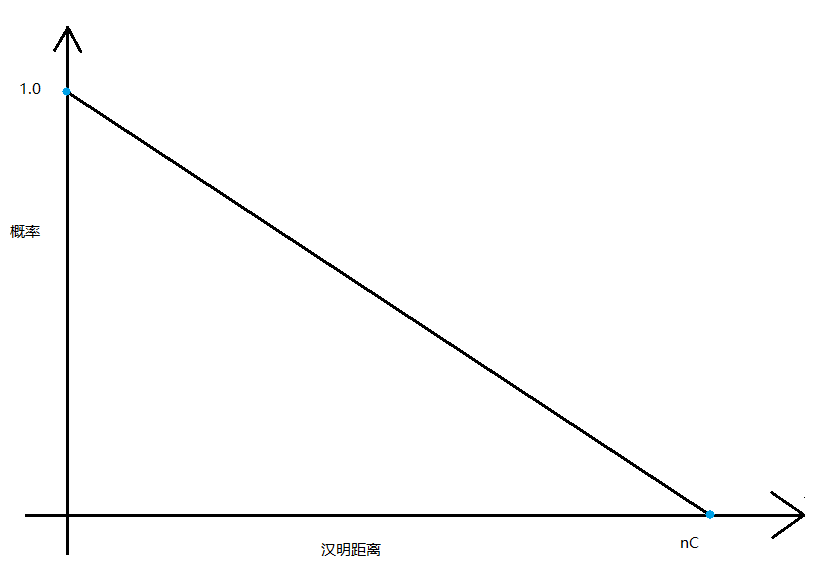## 五. LSH的重要参数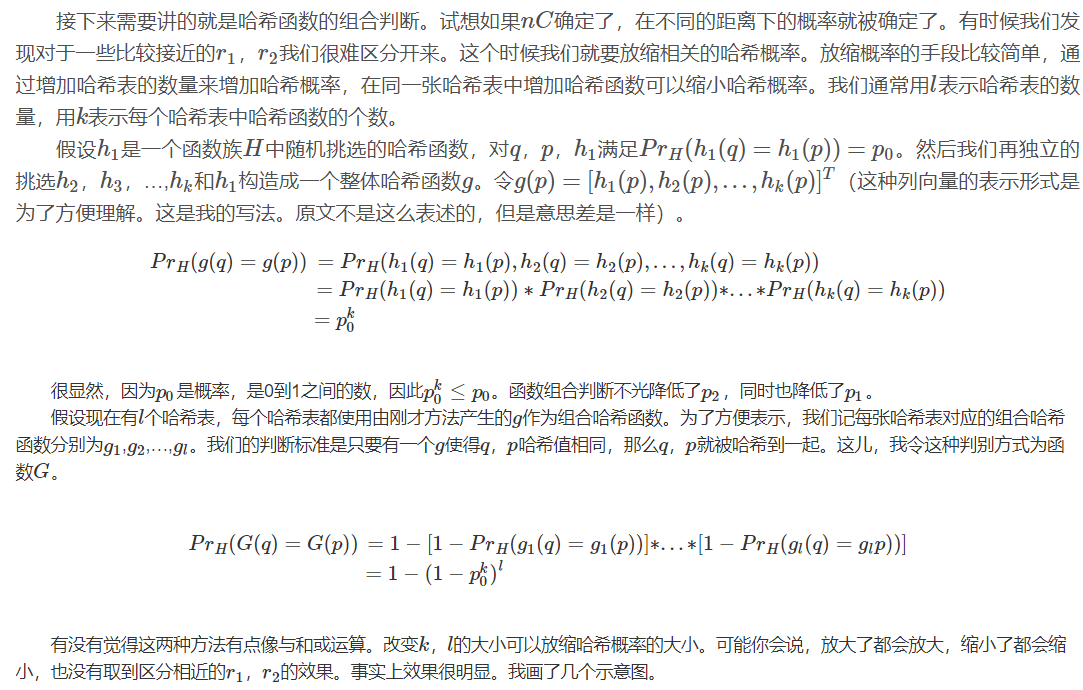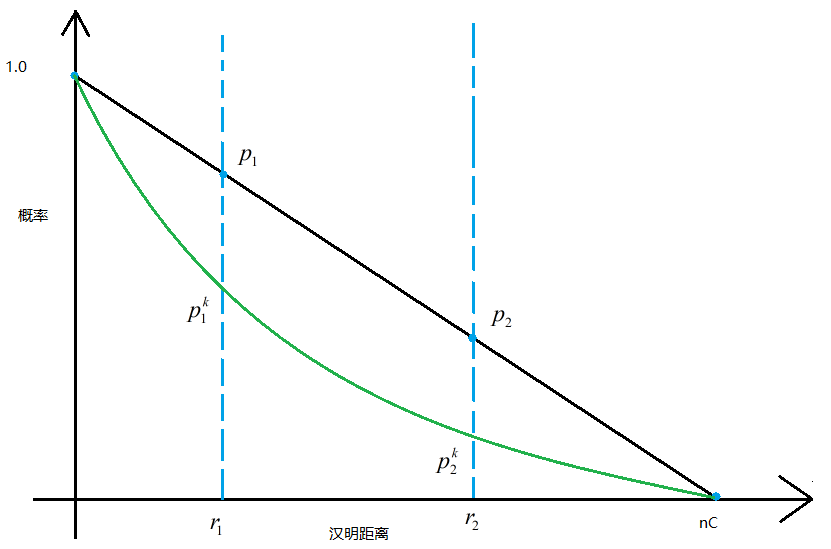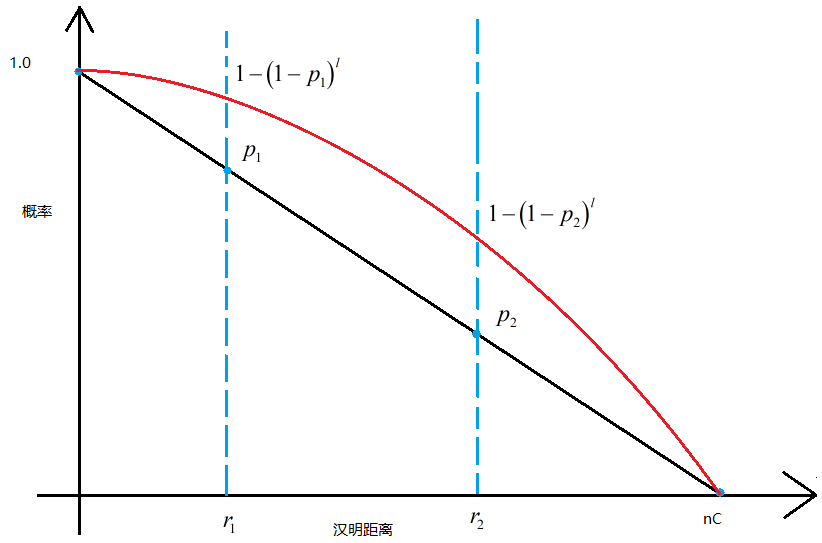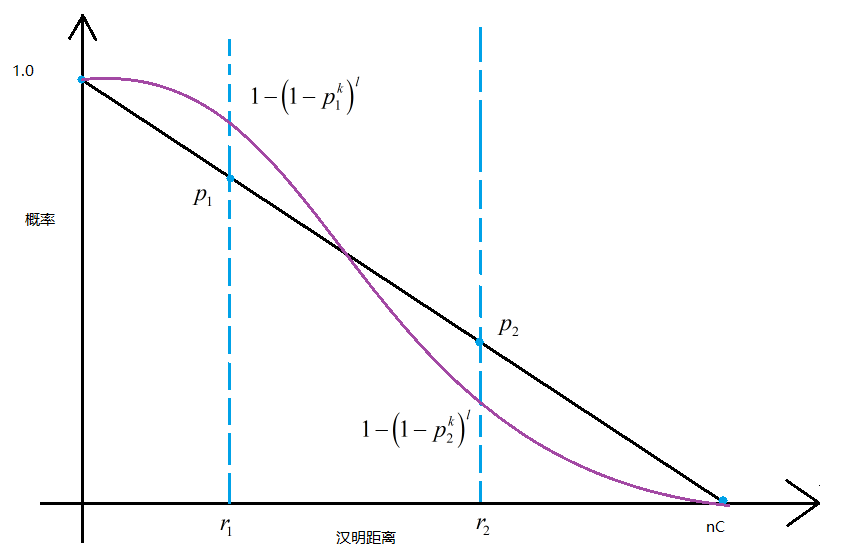A = ( 1 , 1 ) B = ( 2 , 1 ) C = ( 1 , 2 ) D = ( 2 , 2 ) E = ( 4 , 2 ) F = ( 4 , 3 ) \begin{array}{lll} A=(1,1) & B=(2,1) & C=(1,2) \\ D=(2,2) & E=(4,2) & F=(4,3) \end{array}

v ( A ) = 10001000 v ( B ) = 11001000 v ( C ) = 10001100 v ( D ) = 11001100 v ( E ) = 11111100 v ( F ) = 11111110 \begin{array}{l} v(A)=10001000 \\ v(B)=11001000 \\ v(C)=10001100 \\ v(D)=11001100 \\ v(E)=11111100 \\ v(F)=11111110 \end{array}

G G g 1 , g 2 , g 3 g_{1}, g_{2}, g_{3} 构成。每个 g g 由它对应的 h 1 , h 2 h_{1}, h_{2} 构成

g 1 g_{1} 分别抽取第2，4位。
g 2 g_{2} 分别抽取第1，6位。
g 3 g_{3} 分别抽取第3，8位。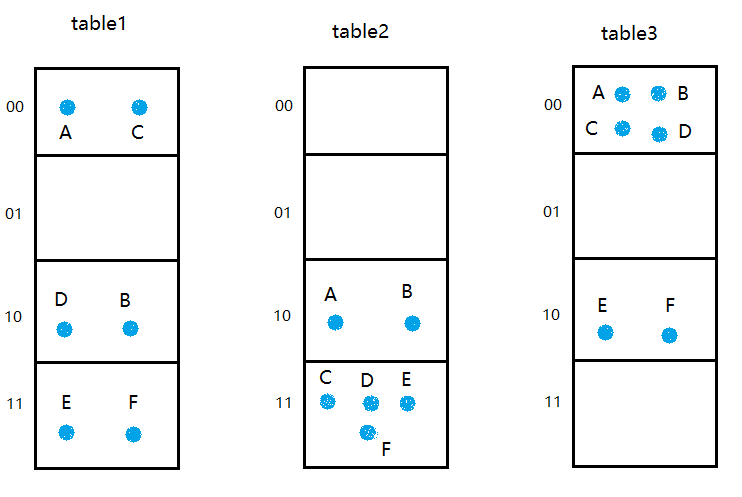M = α n B M=\alpha \frac{n}{B}
α \alpha 是内存利用率。对查询q的近邻点时，我们要搜索所有哈希表至少 c ∗ l c * l 个点。因此磁盘的访问是有上界的，上界便是l。 我们现在来分析下l，k的取值问题。首先我们列出两个事件。 假如我们的哈希函数是满足 ( r 1 , r 2 , p 1 , p 1 ) \left(r_{1}, r_{2}, p_{1}, p_{1}\right) 性的。

P1：如果存在一个点 p ∗ , p ∗ ∈ B ( q , r 1 ) , p^{*}, p^{*} \in B\left(q, r_{1}\right), 至少存在一个 g j g_{j} 满足 g j ( q ) = g j ( p ∗ ) , j = 1 , 2 , … , l o g_{j}(q)=g_{j}\left(p^{*}\right), j=1,2, \ldots, l_{o}

P2： q通过 g , g, 哈希到的块中仅包含 r 2 r_{2} 以外的点的块数小于cl。

## 六、局部敏感哈希规整

1. 近似检测（Near-duplicate detection）：通常运用在网页去重方面。在搜索中往往会遇到内容相似的重复页面，它们中大多是由于网站之间转载造成的。可以对页面计算LSH，通过查找相等或相近的LSH值找到Near-duplicate。
2. 图像、音频检索：通常图像、音频文件都比较大，并且比较起来相对麻烦，我们可以事先对其计算LSH，用作信息指纹，这样可以给定一个文件的LSH值，快速找到与其相等或相近的图像和文件。
3. 聚类：将LSH值作为样本特征，将相同或相近的LSH值的样本合并在一起作为一个类别。

LSH(Location Sensitive Hash),即位置敏感哈希函数。与一般哈希函数不同的是位置敏感性，也就是散列前的相似点经过哈希之后，也能够在一定程度上相似，并且具有一定的概率保证。
LSH的形式化定义可参见前面部分。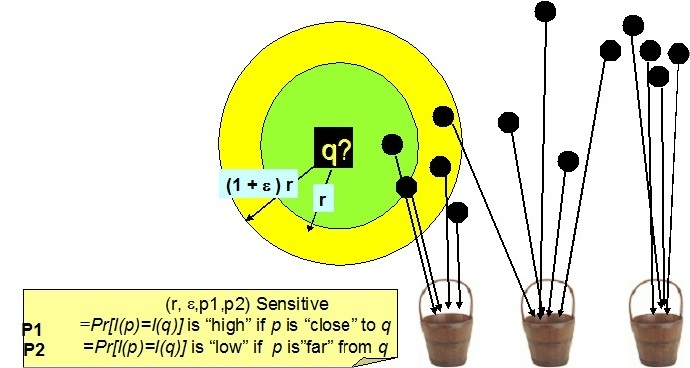LSH的作用:

◆高维下近似查询

1. 高准确性。即返回的结果和线性查找的结果接近。
2. 空间复杂度低。即占用内存空间少。理想状态下，空间复杂度随数据集呈线性增长，但不会远大于数据集的大小。
3. 时间复杂度低。检索的时间复杂度最好为O（1）或O（logN)。
4. 支持高维度。能够较灵活地支持高维数据的检索。

1998年, P.Indy和R.Motwani提出了LSH算法的理论基础。1999 年Gionis A,P.Indy和R.Motwani使用哈希的办法解决高维数据的快速检索问题, 这也是Basic LSH算法的雏形。2004 年, P.Indy 提出了LSH 算法在欧几里德空间(2-范数)下的具体解决办法。同年, 在自然语言处理领域中, Deepak Ravichandran使用二进制向量和快速检索算法改进了Basic LSH 算法, 并将其应用到大规模的名词聚类中, 但改进后的算法时间效率并不理想。

2005 年, Mayank Bawa, Tyson Condie 和Prasanna Ganesan 提出了LSH Forest算法, 该算法使用树形结构代替哈希表, 具有自我校正参数的能力。2006 年, R. Panigrahy用产生近邻查询点的方法提高LSH 空间效率, 但却降低了算法的空间效率。2007年,William Josephson 和Zhe Wang使用多重探测的方法改进了欧几里德空间(2-范数)下的LSH 算法, 同时提高了算法的时间效率和空间效率。

◆分类和聚类

◆数据压缩。如广泛地应用于信号处理及数据压缩等领域的Vector Quantization量子化技术。

### 进入LSH实现部分，将按LSH的发展顺序介绍几种应用广泛的LSH算法。

1, 基于Stable Distribution投影方法
2, 基于随机超平面投影的方法;
3, SimHash;
4, Kernel LSH

1, 基于Stable Distribution投影方法

2008年IEEE Signal Process上有一篇文章Locality-Sensitive Hashing for Finding Nearest Neighbors是一篇较为容易理解的基于Stable Dsitrubution的投影方法的Tutorial, 有兴趣的可以看一下. 其思想在于高维空间中相近的物体，投影（降维）后也相近。基于Stable Distribution的投影LSH，就是产生满足Stable Distribution的分布进行投影，最后将量化后的投影值作为value输出. 更详细的介绍在Alexandr Andoni维护的LSH主页中，理论看起来比较复杂，不过这就是LSH方法的鼻祖啦，缺点显而易见：你需要同时选择两个参数，并且量化后的哈希值是一个整数而不是bit形式的0和1，你还需要再变换一次。如果要应用到实际中，简直让你抓狂。

2, 基于随机超平面投影的方法

1),不需要参数设定
2),是两个向量间的cosine距离，非常适合于文本度量
3),计算后的value值是比特形式的1和0，免去了前面算法的再次变化

3, SimHash

a，将一个f维的向量V初始化为0；f位的二进制数S初始化为0；
b，对每一个特征：用传统的hash算法（究竟是哪种算法并不重要，只要均匀就可以）对该特征产生一个f位的签名b。对i=1到f：

c，如果V的第i个元素大于0，则S的第i位为1，否则为0；
d，输出S作为签名。

4, Kernel LSH

07-03
10-011万+03-174605
03-097572
06-08
01-15
07-07161
06-044920
08-031757